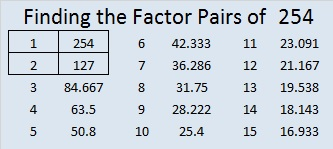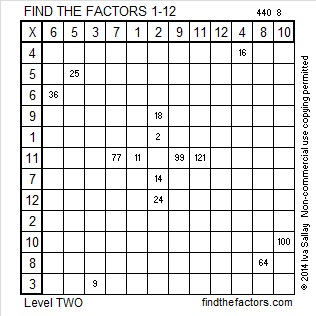# 254 and Level 2

• 254 is a composite number.
• Prime factorization: 254 = 2 x 127
• The exponents in the prime factorization are 1 and 1. Adding one to each and multiplying we get (1 + 1)(1 + 1) = 2 x 2 = 4. Therefore 254 has 4 factors.
• Factors of 254: 1, 2, 127, 254
• Factor pairs: 254 = 1 x 254 or 2 x 127
• 254 has no square factors that allow its square root to be simplified. √254 ≈ 15.937Print the puzzles or type the factors on this excel file: 12 Factors 2014-10-06This site uses Akismet to reduce spam. Learn how your comment data is processed.